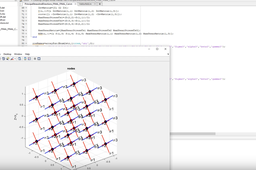# Matlab Tutorial: How to calculate the principal stresses and directions for a nodal gridHow to achieve calculation, plotting of principal stresses and directions for a nodal grid?
This tutorial shows how to achieve this calculation!